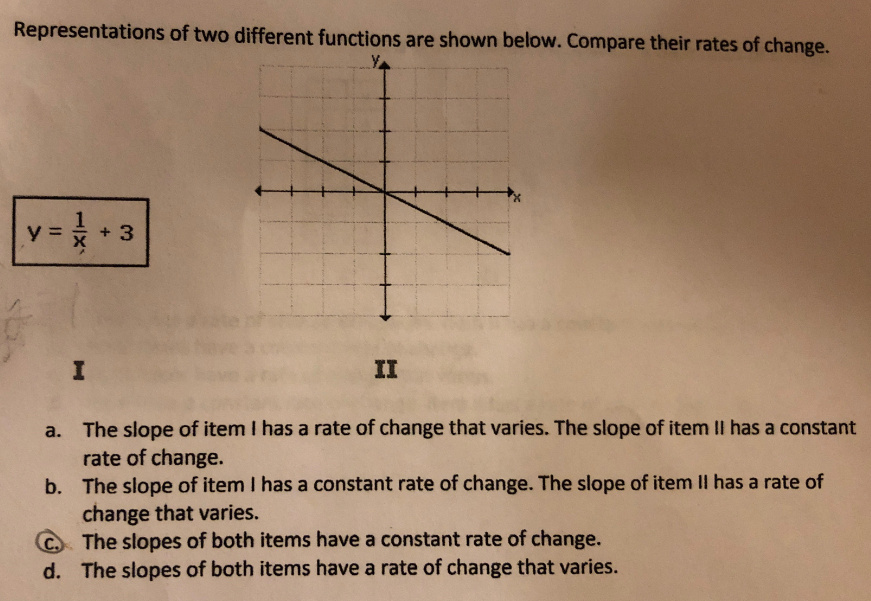# Representations of two different functions are shown below. Compare their rates of change. y =* + 3 II a. The slope of item I has a rate of change that varies. The slope of item II has a constant rate of change. b. The slope of item I has a constant rate of change. The slope of item II has a rate of change that varies. C The slopes of both items have a constant rate of change. d. The slopes of both items have a rate of change that varies.

Questionhelp_outlineImage TranscriptioncloseRepresentations of two different functions are shown below. Compare their rates of change. y =* + 3 II a. The slope of item I has a rate of change that varies. The slope of item II has a constant rate of change. b. The slope of item I has a constant rate of change. The slope of item II has a rate of change that varies. C The slopes of both items have a constant rate of change. d. The slopes of both items have a rate of change that varies. fullscreen

### Want to see this answer and more?

Experts are waiting 24/7 to provide step-by-step solutions in as fast as 30 minutes!*

*Response times may vary by subject and question complexity. Median response time is 34 minutes for paid subscribers and may be longer for promotional offers.
Tagged in
Math
Algebra

### Other